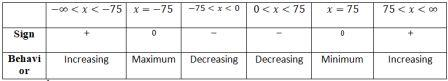# Average Cost. A manufacturer incurs the following cost in producing x rain jackets in one day or...

## Question:

Average Cost. A manufacturer incurs the following cost in producing x rain jackets in one day or 0< x < 200: fixed cost $450; unit production cost ,$30 per jacket; equipment maintenance and repairs , 0.08 x^2 dollars.

(A) What is the average cost C(x) per jacket if x jackets as produced in one day ?

(B) Find the critical number of C (x), the intervals on which the average cost per jacket is decreasing , the intervals on which the average cost per jacket is increasing and the local extreme. P.S.

## Critical Point and Local Extreme

This problem is based on the application of the derivatives. We have been given a scenario and the constraints related to it our aim would be to establish the required functions followed by the use of the derivatives to calculate the critical point followed by the calculation of the local extremes.

According to the given information, there is a fixed cost of $450 and$30 per jacket plus the equipment maintenance and repairs cost {eq}\displaystyle 0.08x^2 {/eq}.

Let us establish the total cost function first, if {eq}\displaystyle x {/eq} jackets:

{eq}\displaystyle \text{Total cost function} = C(x)=0.08x^2+30x+450 {/eq}

A.

The average cost is given as the ratio of total cost and the number of units produced. So,

{eq}\displaystyle \text{Average cost}=\frac{C(x)}{x} {/eq}

{eq}\displaystyle \text{Average cost}=\frac{0.08x^2+30x+450}{x} {/eq}

Average cost is given as:

{eq}\displaystyle \boxed{\displaystyle \text{Average cost}=0.08x+30+\frac{450}{x}} {/eq}

B.

Our total cost function is given as:

{eq}\displaystyle C(x)=0.08x^2+30x+450 {/eq}

Let us find the critical number after finding the first derivative:

{eq}\displaystyle C'(x)=0.16x+30 {/eq}

{eq}\displaystyle C'(x)=0.16x+30=0 {/eq}

{eq}\displaystyle 0.16x+30=0 {/eq}

Thus, the critical point is:

{eq}\displaystyle \boxed{\displaystyle x=-187.5} {/eq}

The average cost function is given as:

{eq}\displaystyle \text{Average cost}=A(x)=0.08x+30+\frac{450}{x} {/eq}

Now our aim is to define the intervals of increase and decrease and find the maximum/minimum of this function.

Let us start off by finding the critical point (at a critical point the function's derivative is either zero or is undefined):

{eq}\displaystyle A(x)=0.08x+30+\frac{450}{x} {/eq}

{eq}\displaystyle A'(x)=0.08-\frac{450}{x^2} {/eq}

{eq}\displaystyle A'(x)=0.08-\frac{450}{x^2}=0 {/eq}

Solving for {eq}\displaystyle x {/eq} we get,

{eq}\displaystyle x=-75, 75 {/eq}

• Be careful that 0 does not come under the domain so the intervals of monotone will split at 0.

The table below summarizes the information derived from the mathematical analysis:So, the:

{eq}\displaystyle \boxed{\displaystyle \text{Intervals of increase}:(-\infty,75)\cup(75,\infty)} {/eq}

{eq}\displaystyle \boxed{\displaystyle \text{Intervals of decrease}:(-75,0)\cup(0,75)} {/eq}

{eq}\displaystyle \boxed{\displaystyle \text{Maximum value}= 18 \text{ at }x=-75} {/eq}

{eq}\displaystyle \boxed{\displaystyle \text{Minimum value}= 42 \text{ at }x=75} {/eq}